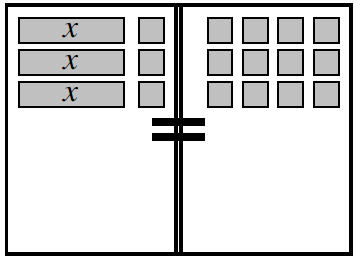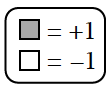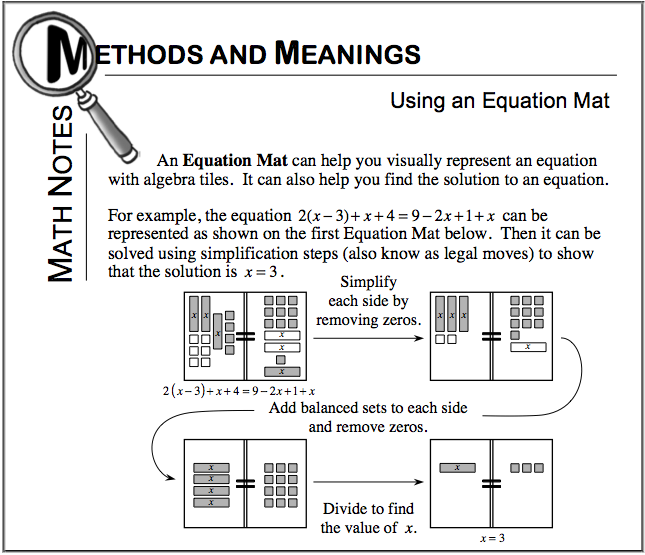### Home > CC2MN > Chapter 6 Unit 6B > Lesson CC2: 6.2.1 > Problem6-54

6-54.Consider the Equation Mat at right.  Homework Help ✎Refer to the Math Notes box below for help.

1. Write the original equation represented.

Count the number of $x$-tiles and unit tiles on each side of the equation, then turn that into an equation.

2. Simplify the tiles on the mat as much as possible. What value of $x$ will make the two expressions equal?

Remove matching sets of tiles from each side.

Now you are left with $3$ $x$-tiles on the left and $9$ unit tiles on the right. Divide each side by $3$ to find the value of $x$.

$x=3$